# AC phase

## AC Electric Circuits

• #### Question 1

If we were to express the series-connected DC voltages as phasors (arrows pointing with a particular length and a particular direction, graphically expressing magnitude and polarity of an electrical signal), how would we draw them in such a way that the total (or resultant) phasors accurately expressed the total voltage of each series-connected pair?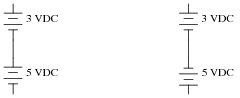If we were to assign angle values to each of these phasors, what would you suggest?

• #### Question 2

Calculate the total voltage of these series-connected voltage sources:• #### Question 3

Using a computer or graphing calculator, plot the sum of these two sine waves: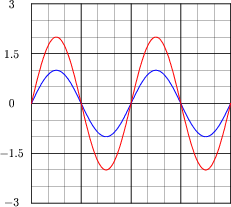What do you suppose the sum of a 1-volt (peak) sine wave and a 2-volt (peak) sine wave will be, if both waves are perfectly in-phase with each other?

Hint: you will need to enter equations into your plotting device that look something like this:

y1 = sin x

y2 = 2 * sin x

y3 = y1 y2

• #### Question 4

Using a computer or graphing calculator, plot the sum of these two sine waves: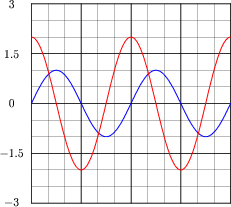Hint: you will need to enter equations into your plotting device that look something like this:

y1 = sin x

y2 = 2 * sin (x 90)

y3 = y1 y2

Note: the second equation assumes your calculator has been set up to calculate trigonometric functions in angle units of degrees rather than radians. If you wish to plot these same waveforms (with the same phase shift shown) using radians as the unit of angle measurement, you must enter the second equation as follows:

y2 = 2 * sin (x 1.5708)

• #### Question 5

Special types of vectors called phasors are often used to depict the magnitude and phase-shifts of sinusoidal AC voltages and currents. Suppose that the following phasors represent the series summation of two AC voltages, one with a magnitude of 3 volts and the other with a magnitude of 4 volts: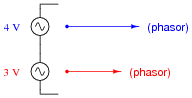Explain what each of the following phasor diagrams represents, in electrical terms: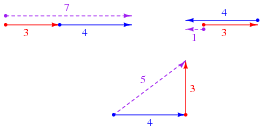Also explain the significance of these sums: that we may obtain three different values of total voltage (7 volts, 1 volt, or 5 volts) from the same series-connected AC voltages. What does this mean for us as we prepare to analyze AC circuits using the rules we learned for DC circuits?

• #### Question 6

When drawing phasor diagrams, there is a standardized orientation for all angles used to ensure consistency between diagrams. This orientation is usually referenced to a set of perpendicular lines, like the x and y axes commonly seen when graphing algebraic functions:The intersection of the two axes is called the origin, and straight horizontal to the right is the definition of zero degrees (0o). Thus, a phasor with a magnitude of 6 and an angle of 0o would look like this on the diagram: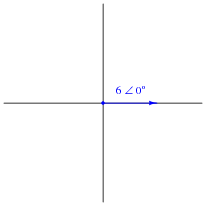Draw a phasor with a magnitude of 10 and an angle of 100 degrees on the above diagram, as well as a phasor with a magnitude of 2 and an angle of -45 degrees. Label what directions 90o, 180o, and 270o would indicate on the same diagram.

• #### Question 7

What does it mean to add two or more phasors together, in a geometric sense? How would one draw a phasor diagram showing the following two phasors added together?• #### Question 8

Determine the sum of these two phasors, and draw a phasor diagram showing their geometric addition:

(4 ∠ 0o) (3 ∠ 90o)

How might a phasor arithmetic problem such as this relate to an AC circuit?

• #### Question 9

Phasors may be symbolically described in two different ways: polar notation and rectangular notation. Explain what each of these notations means, and why either one may adequately describe a phasor.

• #### Question 10

These two phasors are written in a form known as polar notation. Re-write them in rectangular notation:

 4 ∠ 0o =

 3 ∠ 90o =

• #### Question 11

In this graph of two AC voltages, which one is leading and which one is lagging?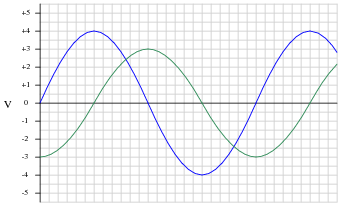If the 4-volt (peak) sine wave is denoted in phasor notation as 4 V ∠ 0o, how should the 3-volt (peak) waveform be denoted? Express your answer in both polar and rectangular forms.

If the 4-volt (peak) sine wave is denoted in phasor notation as 4 V ∠ 90o, how should the 3-volt (peak) waveform be denoted? Express your answer in both polar and rectangular forms.

• #### Question 12

A common feature of oscilloscopes is the X−Y mode, where the vertical and horizontal plot directions are driven by external signals, rather than only the vertical direction being driven by a measured signal and the horizontal being driven by the oscilloscope’s internal sweep circuitry: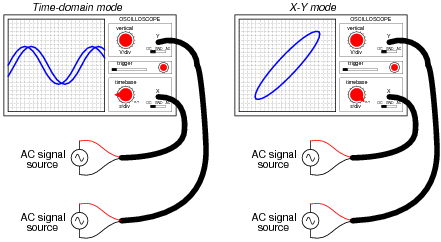The oval pattern shown in the right-hand oscilloscope display of the above illustration is typical for two sinusoidal waveforms of the same frequency, but slightly out of phase with one another. The technical name for this type of X−Y plot is a Lissajous figure.

What should the Lissajous figure look like for two sinusoidal waveforms that are at exactly the same frequency, and exactly the same phase (0 degrees phase shift between the two)? What should the Lissajous figure look like for two sinusoidal waveforms that are exactly 90 degrees out of phase?

A good way to answer each of these questions is to plot the specified waveforms over time on graph paper, then determine their instantaneous amplitudes at equal time intervals, and then determine where that would place the “dot” on the oscilloscope screen at those points in time, in X−Y mode. To help you, I’ll provide two blank oscilloscope displays for you to draw the Lissajous figures on: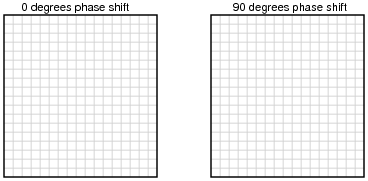• #### Question 13

Lissajous figures, drawn by an oscilloscope, are a powerful tool for visualizing the phase relationship between two waveforms. In fact, there is a mathematical formula for calculating the amount of phase shift between two sinusoidal signals, given a couple of dimensional measurements of the figure on the oscilloscope screen.

The procedure begins with adjusting the vertical and horizontal amplitude controls so that the Lissajous figure is proportional: just as tall as it is wide on the screen (n). Then, we make sure the figure is centered on the screen and we take a measurement of the distance between the x-axis intercept points (m), as such: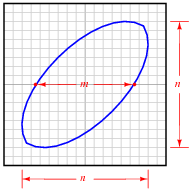Determine what the formula is for calculating the phase shift angle for this circuit, given these dimensions. Hint: the formula is trigonometric! If you don’t know where to begin, recall what the respective Lissajous figures look like for a 0o phase shift and for a 90o phase shift, and work from there.

• #### Question 14

Calculate the amount of phase shift indicated by this Lissajous figure: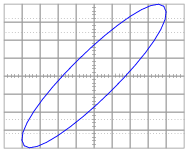• #### Question 15

Calculate the amount of phase shift indicated by this Lissajous figure: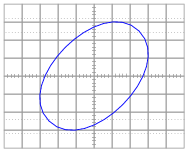• #### Question 16

Calculate the amount of phase shift indicated by this Lissajous figure: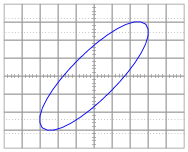• #### Question 17

Calculate the amount of phase shift indicated by this Lissajous figure: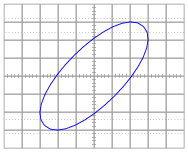• #### Question 18

Suppose two people work together to slide a large box across the floor, one pushing with a force of 400 newtons and the other pulling with a force of 300 newtons: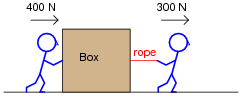The resultant force from these two persons’ efforts on the box will, quite obviously, be the sum of their forces: 700 newtons (to the right).

What if the person pulling decides to change position and push sideways on the box in relation to the first person, so the 400 newton force and the 300 newton force will be perpendicular to each other (the 300 newton force facing into the page, away from you)? What will the resultant force on the box be then?• #### Question 19

In this phasor diagram, determine which phasor is leading and which is lagging the other: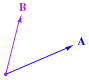• #### Question 20

Is it appropriate to assign a phasor angle to a single AC voltage, all by itself in a circuit?What if there is more than one AC voltage source in a circuit?• #### Question 21

Determine the total voltage in each of these examples, drawing a phasor diagram to show how the total (resultant) voltage geometrically relates to the source voltages in each scenario:• #### Question 22

Before two or more operating alternators (AC generators) may be electrically coupled, they must be brought into synchronization with each other. If two alternators are out of “sync” (or out of phase) with each other, the result will be a large fault current when the disconnect switch is closed.

A simple and effective means of checking for “sync” prior to closing the disconnect switch for an alternator is to have light bulbs connected in parallel with the disconnect switch contacts, like this:What should the alternator operator look for before closing the alternator switch? Do bright lights indicate a condition of being “in-phase” with the bus, or do dim lights indicate this? What does the operator have to do in order to bring an alternator into “phase” with the bus voltage?

Also, describe what the light bulbs would do if the two alternators were spinning at slightly different speeds.

• #### Question 23

Suppose a power plant operator was about to bring this alternator on-line (connect it to the AC bus), and happened to notice that neither one of the synchronizing lights was lit at all. Thinking this to be unusual, the operator calls you to determine if something is wrong with the system. Describe what you would do to troubleshoot this system.• #### Question 24

Shown here are two sine waves of equal frequency, superimposed on the same graph:As accurately as possible, determine the amount of phase shift between the two waves, based on the divisions shown on the graph.

Also, plot a third sine wave that is the sum of the two sine waves shown. Again, do this as accurately as possible, based on the divisions shown on the graph. To give an example of how you might do this, observe the following illustration: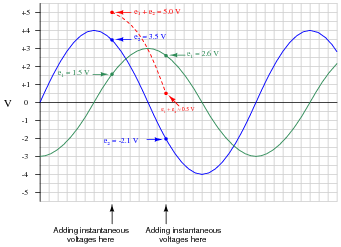What is the peak value of the resultant (sum) sine-wave? How does this compare with the peak values of the two original sine waves?